# How to get a list of unique and distinct values in Excel

This is the final part of the Excel Unique Values series that shows how to get a list of distinct / unique values in column using a formula, and how to tweak that formula for different datasets. You will also learn how to quickly get a distinct list using Excel's Advanced Filter, and how to extract unique rows with Duplicate Remover.

In a couple of recent articles, we discussed different methods to count and find unique values in Excel. If you had a chance to read those tutorials, you already know how to get a unique or distinct list by identifying, filtering, and copying. But that's a bit long, and by far not the only, way to extract unique values in Excel. You can do it much faster by using a special formula, and in a moment I will show you this and a couple of other techniques.

Tip. To quickly get unique values in the latest version of Excel 365 that supports dynamic arrays, use the UNIQUE function.

## How to get unique values in Excel

To avoid any confusion, first off, let's agree on what we call unique values in Excel. Unique values are the values that exist in a list only once. For example: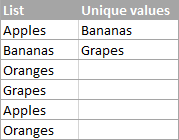To extract a list of unique values in Excel, use one of the following formulas.

Array unique values formula (completed by pressing Ctrl + Shift + Enter):

`=IFERROR(INDEX(\$A\$2:\$A\$10, MATCH(0, COUNTIF(\$B\$1:B1,\$A\$2:\$A\$10) + (COUNTIF(\$A\$2:\$A\$10, \$A\$2:\$A\$10)<>1), 0)), "")`

Regular unique values formula (completed by pressing Enter):

`=IFERROR(INDEX(\$A\$2:\$A\$10, MATCH(0,INDEX(COUNTIF(\$B\$1:B1, \$A\$2:\$A\$10)+(COUNTIF(\$A\$2:\$A\$10, \$A\$2:\$A\$10)<>1),0,0), 0)), "")`

In the above formulas, the following references are used:

• A2:A10 - the source list.
• B1 - the top cell of the unique list minus 1. In this example, we start the unique list in B2, and therefore we supply B1 to the formula (B2-1=B1). If your unique list begins, say, in cell C3, then change \$B\$1:B1 to \$C\$2:C2.

Note. Because the formula references the cell above the first cell of the unique list, which is usually the column header (B1 in this example), make sure your header has a unique name that does not appear anywhere else in the column.

In this example, we are extracting unique names from column A (more precisely from range A2:A20), and the following screenshot demonstrates the array formula in action: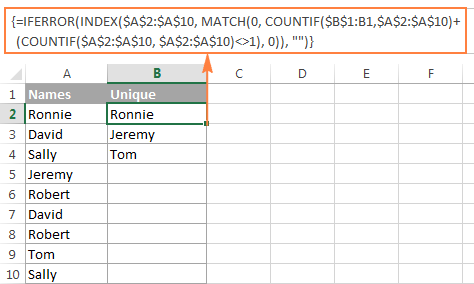The detailed explanation of the formula's logic is provided in a separate section, and here's how to use the formula to extract unique values in your Excel worksheets:

• Tweak one of the formulas according to your dataset.
• Enter the formula in the first cell of the unique list (B2 in this example).
• If you are using the array formula, press Ctrl + Shift + Enter. If you've opted for the regular formula, press the Enter key as usual.
• Copy the formula down as far as needed by dragging the fill handle. Since both unique values formulas are we encapsulated in the IFERROR function, you can copy the formula up to the end of your table, and it won't clutter your data with any errors no matter how few unique values have been extracted.

## How to get distinct values in Excel (unique + 1st duplicate occurrences)

As you may have already guessed from the heading of this section, distinct values in Excel are all different values in a list, i.e. unique values and first instances of duplicate values. For example: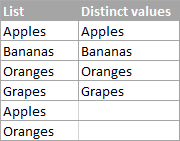To get a distinct list in Excel, use the following formulas.

Array distinct formula (requires pressing Ctrl + Shift + Enter):

`=IFERROR(INDEX(\$A\$2:\$A\$10, MATCH(0, COUNTIF(\$B\$1:B1, \$A\$2:\$A\$10), 0)), "")`

Regular distinct formula:

`=IFERROR(INDEX(\$A\$2:\$A\$10, MATCH(0, INDEX(COUNTIF(\$B\$1:B1, \$A\$2:\$A\$10), 0, 0), 0)), "")`

Where:

• A2:A10 is the source list.
• B1 is the cell above the first cell of the distinct list. In this example, the distinct list begins in cell B2 (it's the first cell where you enter the formula), so you reference B1.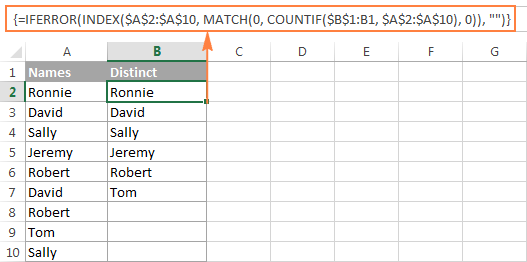### Extract distinct values in a column ignoring blank cells

If your source list contains any blank cells, the distinct formula we've just discussed would return a zero for each empty row, which might be a problem. To fix this, improve the formula a bit further:

Array formula to extract distinct values excluding blanks:

`=IFERROR(INDEX(\$A\$2:\$A\$10, MATCH(0, COUNTIF(\$B\$1:B1, \$A\$2:\$A\$10&"") + IF(\$A\$2:\$A\$10="",1,0), 0)), "")`

### Get a list of distinct text values ignoring numbers and blanks

In a similar manner, you can get a list of distinct values excluding empty cells and cells with numbers:

`=IFERROR(INDEX(\$A\$2:\$A\$10, MATCH(0, COUNTIF(\$B\$1:B1, \$A\$2:\$A\$10&"") + IF(ISTEXT(\$A\$2:\$A\$10)=FALSE,1,0), 0)), "")`

As a quick reminder, in the above formulas, A2:A10 is the source list, and B1 is cell right above the first cell of the distinct list.

The following screenshot shows the result of both formulas: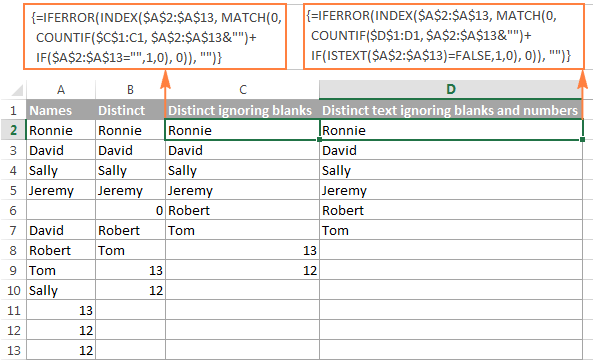### How to extract case-sensitive distinct values in Excel

When working with case-sensitive data such as passwords, user names or file names, you may need to get a list of case-sensitive distinct values. For this, use the following array formula, where A2:A10 is the source list, and B1 is the cell above the first cell of the distinct list:

Array formula to get case-sensitive distinct values (requires pressing Ctrl + Shift + Enter)

`=IFERROR(INDEX(\$A\$2:\$A\$10, MATCH(0, FREQUENCY(IF(EXACT(\$A\$2:\$A\$10,TRANSPOSE(\$B\$1:B1)), MATCH(ROW(\$A\$2:\$A\$10), ROW(\$A\$2:\$A\$10)), ""), MATCH(ROW(\$A\$2:\$A\$10), ROW(\$A\$2:\$A\$10))), 0)), "")`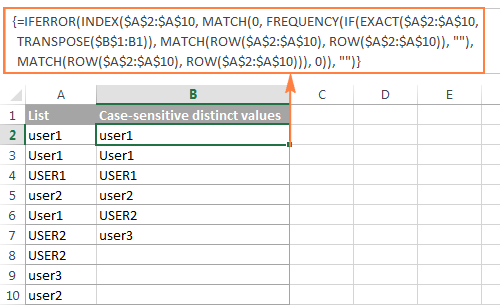## How the unique / distinct formula works

This section is written especially for those curious and thoughtful Excel users who not only want to know the formula but fully understand its nuts and bolts.

It goes without saying that the formulas to extract unique and distinct values in Excel are neither trivial nor straightforward. But having a closer look, you may notice that all the formulas are based on the same approach - using INDEX/MATCH in combination with COUNTIF, or COUNTIF + IF functions.

For our in-depth analysis, let's use the array formula that extracts a list of distinct values because all other formulas discussed in this tutorial are improvements or variations of this basic one:

`=IFERROR(INDEX(\$A\$2:\$A\$10, MATCH(0, COUNTIF(\$B\$1:B1, \$A\$2:\$A\$10), 0)), "")`For starters, let's cast away the obvious IFERROR function, which is used with a single purpose to eliminate #N/A errors when the number of cells where you've copied the formula exceeds the number of distinct values in the source list.

And now, let's break down the core part of our distinct formula:

1. COUNTIF(range, criteria) returns the number of cells within a range that meet a specified condition.

In this example, COUNTIF(\$B\$1:B1, \$A\$2:\$A\$10) returns an array of 1's and 0's based on whether any of the values of the source list (\$A\$2:\$A\$10) appears somewhere in the distinct list (\$B\$1:B1). If the value is found, the formula returns 1, otherwise - 0.

In particular, in cell B2, COUNTIF(\$B\$1:B1, \$A\$2:\$A\$10) becomes:

`COUNTIF("Distinct", {"Ronnie"; "David"; "Sally"; "Jeremy"; "Robert"; "David"; "Robert"; "Tom"; "Sally"})`

and returns:

`{0;0;0;0;0;0;0;0;0}`

because none of the items of the source list (criteria) appears in the range where the function looks for a match. In this case, range (\$B\$1:B1) consists of a single item - "Distinct".

2. `MATCH(lookup_value, lookup_array, [match_type])` returns the relative position of the lookup value in the array.
In this example, the lookup_value is 0, and consequently:

`MATCH(0,COUNTIF(\$B\$1:B1, \$A\$2:\$A\$10), 0)`

turns into:

`MATCH(0, {0;0;0;0;0;0;0;0;0},0)`

and returns 1

because our MATCH function gets the first value that is exactly equal to the lookup value (as you remember, the lookup value is 0).

3. INDEX(array, row_num, [column_num]) returns a value in an array based on the specified row and (optionally) column numbers.

In this example, INDEX(\$A\$2:\$A\$10, 1)

becomes:

`INDEX({"Ronnie"; "David"; "Sally"; "Jeremy"; "Robert"; "David"; "Robert"; "Tom"; "Sally"}, 1)`

and returns "Ronnie".

When the formula is copied down the column, the distinct list (\$B\$1:B1) expands because the second cell reference (B1) is a relative reference that changes according to the relative position of the cell where the formula moves.

So, when copied to cell B3, COUNTIF(\$B\$1:B1, \$A\$2:\$A\$10) changes to COUNTIF(\$B\$1:B2, \$A\$2:\$A\$10), and becomes:

`COUNTIF({"Distinct";"Ronnie"}, {"Ronnie"; "David"; "Sally"; "Jeremy"; "Robert"; "David"; "Robert"; "Tom"; "Sally"}), 0)), "")`

and returns:

`{1;0;0;0;0;0;0;0;0}`

because one "Ronnie" is found in range \$B\$1:B2.

And then, MATCH(0,{1;0;0;0;0;0;0;0;0},0) returns 2, because 2 is the relative position of the first 0 in the array.

And finally, `INDEX(\$A\$2:\$A\$10, 2)` returns the value from the 2nd row, which is "David".

Tip. For better understanding of the formula's logic, you can select different parts of the formula in the formula bar and press F9 to see what a selected part evaluates to: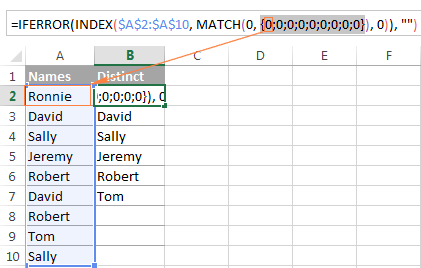If you still have difficulties figuring out the formula, you can check out the following tutorial for the detailed explanation of how the INDEX/MATCH liaison works: INDEX & MATCH as a better alternative to Excel VLOOKUP.

As already mentioned, the other formulas discussed in this tutorial are based on the same logic, with just a few modifications:

Unique values formula - contains one more COUNTIF function that excludes from the unique list all items that appear in the source list more than once: `COUNTIF(\$A\$2:\$A\$10, \$A\$2:\$A\$10)<>1`.

Distinct values formula ignoring blanks - here you add an IF function that prevents blank cells from being added to the distinct list: `IF(\$A\$2:\$A\$13="",1,0)`.

Distinct text values formula ignoring numbers - you use the ISTEXT function to check whether a value is text, and the IF function to dismiss all other value types, including blank cells: `IF(ISTEXT(\$A\$2:\$A\$13)=FALSE,1,0)`.

## Extract distinct values from a column with Excel's Advanced Filter

If you don't want to waste time on figuring out the arcane twists of the distinct value formulas, you can quickly get a list of distinct values by using the Advanced Filter. The detailed steps follow below.

1. Select the column of data from which you want to extract distinct values.
2. Switch to the Data tab > Sort & Filter group, and click the Advanced button: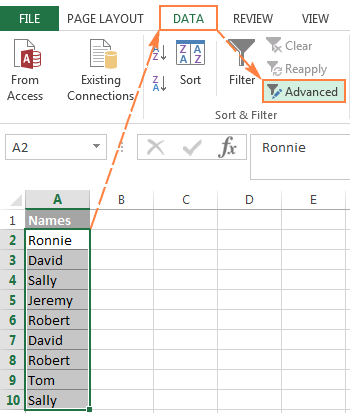3. In the Advanced Filter dialog box, select the following options:
• Check Copy to another location radio button.
• In the List range box, verify that the source range is displayed correctly.
• In the Copy to box, enter the topmost cell of the destination range. Please keep in mind that you can copy the filtered data only to the active sheet.
• Select the Unique records only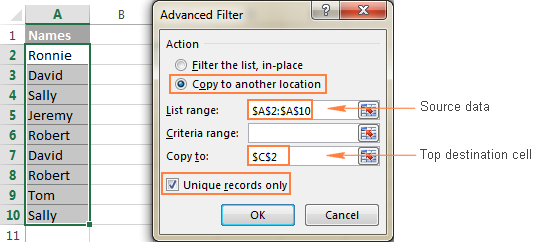4. Finally, click the OK button and check the result: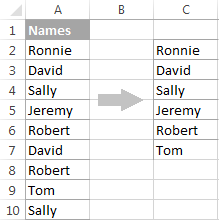Please pay attention that although the Advanced Filter's option is named "Unique records only", it extracts distinct values, i.e. unique values and 1st occurrences of duplicate values.

## Extract unique and distinct rows with Duplicate Remover

In the final part of this tutorial, let me show you our own solution to find and extract distinct and unique values in Excel sheets. This solution combines the versatility of Excel formulas and simplicity of the advanced filter. In addition, it provides a couple of unique features such as:

• Find and extract unique / distinct rows based on values in one or more columns.
• Find, highlight, and copy unique values to any other location, in the same or different workbook.

And now, let's see the Duplicate Remover tool in action.

Supposing you have a summary table created by consolidating data from several other tables. Obviously, that summary table contains a lot of duplicate rows and your task is to extract unique rows that appear in the table only once, or distinct rows including unique and 1st duplicate occurrences. Either way, with the Duplicate Remover add-in the job is done in 5 quick steps.

1. Select any cell within your source table and click the Duplicate Remover button on the Ablebits Data tab, in the Dedupe group.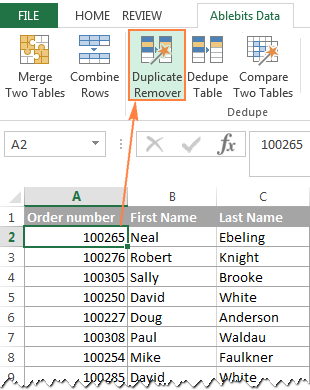The Duplicate Remover wizard will run and select the entire table. So, just click Next to proceed to the next step.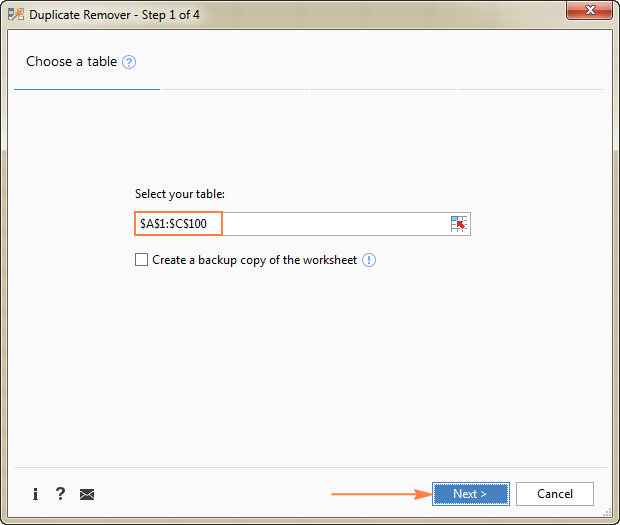2. Select the value type you want to find, and click Next:
• Unique
• Unique +1st occurrences (distinct)

In this example, we aim to extract unique rows that appear in the source table only once, so we select the Unique option: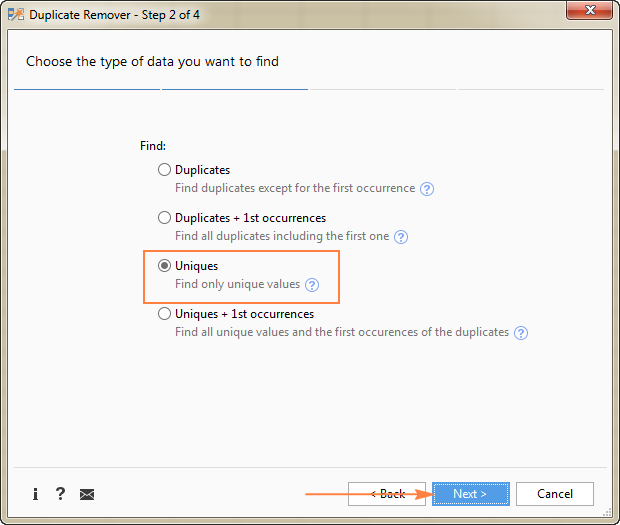Tip. As you can see in the above screenshot, there are also 2 options for duplicate values, just keep it in mind if you need to dedupe some other worksheet.

3. Select one or more columns to be checked for unique values.

In this example, we want to find unique rows based on values in all 3 columns (Order number, First name and Last name), therefore we select all.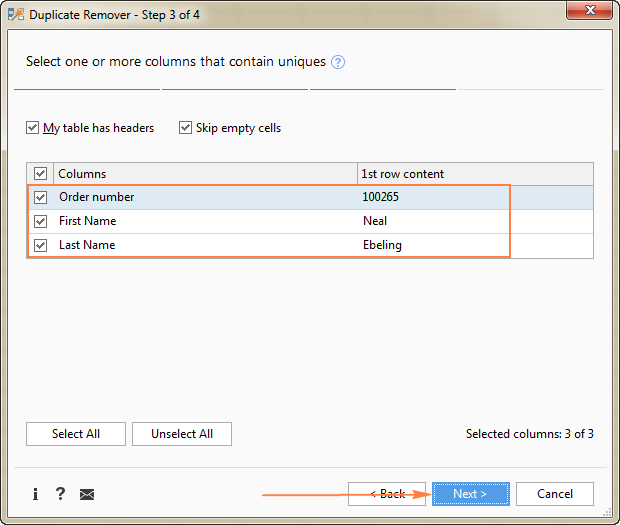4. Choose the action to perform on the found unique values. The following options are available to you:
• Highlight unique values
• Select unique values
• Identify in a status column
• Copy to another location

Because we are extracting unique rows, select Copy to another location, and then specify where exactly you want to copy them - active sheet (select the Custom location option, and specify the top cell of the destination range), new worksheet or new workbook.

In this example, let's opt for the new sheet: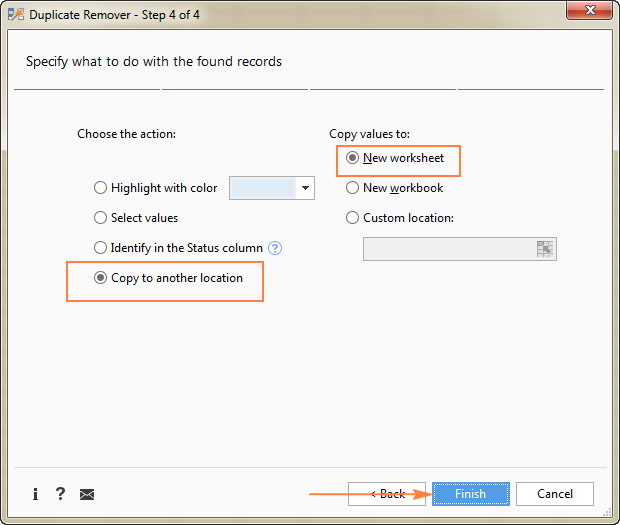5. Click the Finish button, and you are done!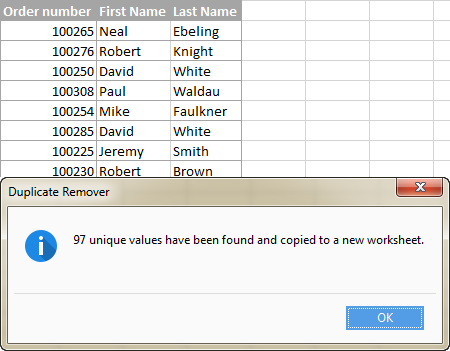Liked this quick and simple way to get a list of unique values or rows in Excel? If so, I encourage you to download an evaluation version below and give it a try. Duplicate Remover as well as all other time-saving tools that we have are included with Ultimate Suite for Excel.

Find Unique Values in Excel - sample workbook (.xlsx file)
Ultimate Suite - evaluation version (.exe file)

## You may also be interested in

1. IN my case I've got a sheet containing a list of appointments between sales people and clients, and I'm trying to count the number of clients each sales person has. To this end, I've used your list distinct values formula to create a list in a column of each sales person, but in a row beside those, I'm trying to create a horizontal list of all distinct clients that sales person has seen. How would I adapt the formula to generate that list of clients?
Thanks for any help!

• Hello!
You can find the examples and detailed instructions here: Get a list of unique values based on criteria.
To write the result in one cell, you can use the TEXTJOIN function:

=TEXTJOIN(", ",TRUE,UNIQUE(FILTER(A2:A10,C2:C10=F1),FALSE))

2. The distinct value formula is spot on. But is there a way to keep those distinct values within the row its mimicking?
For example
Apples was in row 15 and the formula moves apples to row 1. I need apples to stay in row 15 but still identified as a "distinct" value.

3. Hi
Many thanks for this article. I have data like the below in sheet TZ. In another sheet, I am listing the unique dates under column J.

Date | Amount | CODE
(column B)
------- ----------- ---------
15-JUL-2021 25000 HIN
15-JUL-2021 22000 HIN
13-JUL-2021 42000 TIN
12-JUL-2021 37000 HIN
07-JUL-2021 26000 PIN
06-JUL-2021 14000 HIN

From this list, I want to extract distinct values of date corresponding to a code HIN alone.

=IFERROR(INDEX(TZ!\$B\$2:TZ!\$B\$1746, MATCH(0, IF(ISBLANK(TZ!\$B\$2:TZ!\$B\$1746),1,COUNTIFS(\$J\$1:J1, TZ!\$B\$2:TZ!\$B\$1746) ), 0)), "")

4. Hi

I have a list of historical orders as follows

Harry Skirt1
Harry Skirt2
Harry Skirt2
Harry Tie1
Harry Tie1
Harry Skirt1
Tony Trouser1
Tony Skirt1
Tony Trouser1
Tony Skirt1

What I want to be able to do is type customer name into a box and list the products they took (removing duplications)

So in a cell I type (or choose from a list) Harry

That gives me:
Skirt1
Skirt2
Tie1

How do I do that - I have a lot of data as its listed by SKU (style / colour / size) so the formula in the instructions took a long time to load up

Appreciate any help guys

5. Hi Everyone,

I'm having trouble that the unique function is still returning duplicates for me. I've tried both the UNIQUE function and other code using the COUNTIF function to do the same thing - same results. I assume the problem is somehow related to formatting. Here's what I'm trying to do:

I'm breaking a list of numbers in column F into:
(Column G) # Multiples of a certain number (in G2): =ROUNDDOWN(F7/\$G\$2,0)
(Column H) # The remainder: =F7-\$G\$2*G7

I then want to find all the unique values from the remainder column H (this is column J), but it's returning multiples of some numbers. Note that my data starts at row 7, hence G2 is unrelated to the # Multiples data.

I've narrowed it down a little to a concise example:

G2 = 3.6

F G H J

3.4 0 3.4 3.4
25 6 3.4 3.4

Why is the unique function not excluding the second instance of 3.4 in column H?

• Hello!
What formula are you using in column J? In what range do you want to find unique values?

6. Hello,

When I do the regular distinct formula:
=IFERROR(INDEX(\$A\$2:\$A\$10, MATCH(0, INDEX(COUNTIF(\$B\$1:B1, \$A\$2:\$A\$10), 0, 0), 0)), "")

Excel gives me 0 but when I click Ctrl + F9, It gives me :
{"Aportes"}
Aportes is the unique value I would like to see but it gives 0.

Can someone help me?

• Hi,
I have not found an error in this formula. Without seeing your data it is difficult to give you any advice. It works for me correctly. You may not have entered it as an array formula.

7. Looking for a formula to fetch results as below
Source Data:

Sub roll no marks
maths 1 8
Eng 2 9
maths 3 7
Eng 4 4
Eng 1 5
maths 2 3
maths 4 7
Eng 3 6

Results Needed:

Roll no Maths Eng
1 8 5
2 3 9
3 7 6
4 7 4

8. Hi Am looking for the below formula

A=1
B=2 like wise i have so many data.

My required is AB = 12 and BA=21 how to put formula for this?

A 1 A 1
B 2 BG 27
C 3
D 4
E 5
F 6
G 7
H 8
I 9

9. Thanks for the quick replay Alexander!

When trying that array formula including entering with Ctrl + Shift + Enter, it pops up an error message window saying, "That function isn't valid." After hitting "OK" to acknowledge the error, it highlights the word "FILTER" in the formula. Perhaps the syntax is not correct...? Any ideas?

Thanks for your help to resolve!

10. Hi,

How do I modify the formula below if I have another criteria column:

=IFERROR(INDEX(\$A\$8:\$A\$8, MATCH(0, INDEX(COUNTIF(\$C\$1:C1, \$A\$2:\$A\$8), 0, 0), 0)), "")

For example:

A1 B1
Pete C
Betty C
Frank C
Mary O
Frank O
Mary O
Suzy O

I want the formula to look only at rows with "O" and return distinct names.

Result:

C1
Mary
Frank
Suzy

Your help is much appreciated! Thank you!

• Hello!

=IFERROR(INDEX(FILTER(A2:A10,B2:B10="O"), MATCH(0, COUNTIF(\$C\$1:C1, FILTER(A2:A10,B2:B10="O")), 0)), "")

This is an array formula and it needs to be entered via Ctrl + Shift + Enter, not just Enter.

11. Great Article-

I have a follow-up question I cannot figure out. I want to create a list of unique values from another tab that dynamically updates, only I want the new values to always paste/fill in at the bottom of the unique value list. When I change the data source by copying in new data, sometimes new unique values appear in the middle or top of the data set and then these values appear in the middle of my output list. I cannot have this occur as I need the list values to stay in the same rows so that they are matched with some manually input information that goes with them, rather than moving around without the accompanying values in other columns moving with them.

12. Hi,
How to How to get distinct values in Excel (unique + 1st duplicate occurrences), based in a cell reference
for example
Column A Column B
A Apple
B Banana
A pineapple
C Orange
A Apple

I want a list of distinct values based in column A i.e if A, then
Apple
Pine Apple
if B
Banana

• Hello!
Study the section in this article, "How to get distinct values in Excel." If you have specific questions after that, I will try to answer them.

13. Hi
I am trying to do a lookup from one sheet within multiple rows and columns. Customer, Part number will have different qty breaks with different prices.Rows have duplicate part numbers and customer, possible price.
Thanks
How would you recommend the easiest way?

14. Hi
I am trying to do a lookup from one sheet within multiple rows and columns.
Customer, Part number will have different qty breaks with different prices.
Rows have duplicate part numbers and customer, possible price.
Thanks

How would you recommend the easiest way?

15. I have two sheets
First sheet has 470 records, some duplicates.
Second sheet has 1000 records, some duplicates.
I want to find all unique values on sheet one that are NOT on sheet two.
So, any value found in sheet two that matches on sheet one will not be shown. I only want unique values from sheet one. If there are duplicates within sheet one that are not on sheet two they should remain after filtering.

16. Can I get a formula to remove duplicates and blanks when the data is displayed in a row. I tried to make some changes in the formula which is mentioned for data placed in a column, but couldn't get the desired results.

• Hello Keshav!
If the list of values is on the line (for example, A2:P2), then the list of unique values can be obtained using the formula in cell S2.
Regular unique values formula (completed by pressing Enter):

=IFERROR(INDEX(\$A\$2:\$P\$2, MATCH(0,INDEX(COUNTIF(\$S\$1:S1, \$A\$2:\$P\$2) + (COUNTIF(\$A\$2:\$P\$2, \$A\$2:\$P\$2)<>1),0,0), 0)), "")

In cell U2, we write the formula of the array (completed by pressing Ctrl + Shift + Enter):

=IFERROR(INDEX(\$A\$2:\$P\$2, MATCH(0, COUNTIF(\$U\$1:U1,\$A\$2:\$P\$2) + (COUNTIF(\$A\$2:\$P\$2, \$A\$2:\$P\$2)<>1), 0)), "")

After that you can copy this formula down along the column.

17. Regarding the formula +IFERROR(INDEX(\$A\$2:\$A\$10, MATCH(0, COUNTIF(\$B\$1:B1, \$A\$2:\$A\$10), 0)), ""), I only getting the first value as it takes B1 and does not update to b2 b3 and so on when I use it. The array is not working.

• Hello,
You write this formula in cell B2.This is an array formula and it needs to be entered via Ctrl + Shift + Enter, not just Enter. You have not done so. After that you can copy this formula down along the column.

18. Hello,
Great piece of knowledge for a novice like me.
2. By applying the formula =IFERROR(INDEX(\$A\$2:\$A\$10, MATCH(0, INDEX(COUNTIF(\$B\$1:B1, \$A\$2:\$A\$10), 0, 0), 0)), "") the result is in the same order as was in original column, (a)It should come out sorted alphabetically (b)with other data in the row. Please provide formula for this.
It will be great help. God Bless You.

19. =IFERROR(INDEX(\$A\$2:\$A\$10, MATCH(0, INDEX(COUNTIF(\$B\$1:B1, \$A\$2:\$A\$10), 0, 0), 0)), "") works but one needs to figure out how many distinct values are going to exist in order to copy and paste the formula that many times. Hence, if there are 10 distinct values then one copies and paste the formula 9 times. Is there a way for one does not need to know how many distinct values are going to exist?

Thank you

• Hello!
To calculate the number of unique values in a data range, use the formula

=SUMPRODUCT(COUNTIF(A2:A20,A2:A20)^(2*ISBLANK(A2:A20)-1))

I hope this will help, otherwise please do not hesitate to contact me anytime.

20. I have a list of employees and each has a unique job category. Based on their job category, I want a unique list of job competencies to show up to select from a pull down list that are only available for that job category.

• Hello!
This link has detailed instructions on our blog.

21. Hi, Thanks for this wonderful tutorial. I am having one issue with dates. I am trying to find unique dates but for blanks it is showing 00/01/1900 which is not desirable. can you suggest how to remove this.

22. I have a list of employees with their work dates in a given year. I want to insert which period they fall in: a date from 15th to 14th of following month. So if the date is 01/05/2020 it should fall in the period 12/15/2019-01/14/2020. What formula would do this?
Employee Work Date Hours PERIOD
John 01/05/2020 2 12/15/2019-01/14/2020
John 01/15/2020 2 01/15/2020-02/14/2020
John 02/05/2020 2 01/15/2020-02/14/2020
John 03/06/2020 2 02/15/2020-03/14/2020

23. Hi,
Is any way to auto up-date next Tab with specified category in ascending order in a row addition/filling data......!
If in Sheet1, we add a Row with distinct Name 'A" or "B" in column,should auto up-date in next/assigned Tab with same Name "A" or "B" like VLOOKUP but if I apply VLOOKUP, that collect /repeat 1st value.
Example:-
Sheet-1
Team PO No Date Status
A Akbar 3-Aug-19 Close
A Babar 3-Aug-19 Close
A Masood 3-Aug-19 Close
A Zara 3-Aug-19 Close
B Mehmood 18-Jan-19 Close
B Ali 25-May-19 Close
B Hina 17-Oct-19 Running

New Tab
Team PO No Date Status
B Mehmood 18-Jan-19 Close
A Akbar 3-Aug-19 Close
A Akbar 3-Aug-19 Close
A Akbar 3-Aug-19 Close
B Mehmood 18-Jan-19 Close
B Mehmood 18-Jan-19 Close
A Akbar 3-Aug-19 Close
Thanks

24. Is it possible to extra distinct collumned data across a row?
If so can you please share a simple formula to use?

25. Is there a way to add a criteria on this formula? I want to execute this formula if it meets a certain criteria from a column? e.g
=IF(A:A="Criteria",(INDEX(\$A\$2:\$A\$10, MATCH(0, COUNTIF(\$B\$1:B1,\$A\$2:\$A\$10) + (COUNTIF(\$A\$2:\$A\$10, \$A\$2:\$A\$10)1), 0)), "")

=IFERROR(INDEX(\$A\$2:\$A\$10, MATCH(0, COUNTIF(\$B\$1:B1,\$A\$2:\$A\$10) + (COUNTIF(\$A\$2:\$A\$10, \$A\$2:\$A\$10)1), 0)), "")

26. How generate list from range in for next column like
123456789101 to 123456789104
123456789108 to 123456789111
The results should be like
123456789101
123456789102
123456789103
123456789104
123456789108
123456789109
123456789110
123456789111

27. Thank You!!

28. @Svetlana Cheusheva thanks a lot. it worked well for me.

29. Great formula to extract the unique values of any list. Well done, I would have not been able to figure it out how to do it on my own. Cheers!

30. hi there
in sheet one I have
in column A the animals Gender
in column B the animals species
in column C the animals age
there are several other column's with weight, colour and other details
I have sorted my data by column B then by C
so if there are 15 cats at the top of the sheet followed by 11 dog and then 3 birds and then 14 rabbits
I would like to take the first 10 rows of each species and copy them into sheet 2
regards leigh

31. Hi there,
Could you please explain that why we are using, and what its meaning that using "zero" value either as match formula's "lookup value", and index formula's "row number" and "column number" ?
I am referring to this:
=IFERROR(INDEX(\$A\$2:\$A\$10, MATCH(0,INDEX(COUNTIF(\$B\$1:B1, \$A\$2:\$A\$10)+(COUNTIF(\$A\$2:\$A\$10, \$A\$2:\$A\$10)1),0,0), 0)), "")

Thank you.

32. Step 1: Use Google Sheets
Step 2: =unique(range)
Step 3: Profit

33. Hi,
I want to select unique entries from a range of cells. I tried this formula and it shows "0" as result.
My range is A3:F12 and i am trying to get the results starting in A15(formula cell). I used the following formula. Please assist.
=IFERROR(INDEX(\$A\$3:\$F\$12,MATCH(0,COUNTIF(\$A\$15:A15,\$A\$3:\$F\$12)+(COUNTIF(\$A\$15:A15,\$A\$3:\$F\$12)1),0)),"")
Regards,
Sunny

34. Column A Column B Desired result
Class Code Stipend Class Code Amount Count
J7-288-001 1500 J7-288-001 1500 3
J7-288-001 1300 J7-288-001 1300 2
J7-288-001 1500
J7-288-001 1300
J7-288-001 1500

35. Hello!
Thanks for a great article. I read it with interest and built a list of unique names from the original list of duplicates using the array formula. My issue is that when I add more non-unique entries to the original list, the new list changes order. This is unhelpful as I use this new list in a table that has vlookup references.
Any ideas why the new list changes the order of the names?
Thanks!
Paul

36. Hi!
Is there a way to make the locked rows in the 'unique + 1st duplicate' example dependant on another column? That way I am able to do this procedure for multiple unique rows.

Thank you!

37. He genius
Anyone know to extract distinct value between 2 dates and 1 criterion. Criterion is either number or mixed. Example my unique value Column A, Date 1 Column B Date 2 Column & my creteria is column C. How to extract unique value in column A between 2 dates and base on criteria C?

38. I tried to get the "list of distinct text values ignoring numbers and blanks" to work in a sheet I was working on, to not avail. Then I copied the example and the formulas given and it would not work as shown. No error messages, just nothing in cells. The function result show the first instance in the dialog box, but not the cell, then nothing below the cell.

39. Thanks alot

40. Hi Svetlana,
It's a great post; well explained. thanks.

A question for you:
Is it possible to use the INDEX formula for an Excel table? I converted your Excel data to a table (Table1) and used the following formula but it does not work:
However, VLOOKUP works but as expected it only gives the first occurrence of the matching field:
=VLOOKUP(D\$2, Table1,2,FALSE)
Any ideas? Many thanks.
Abbas

41. Hello,

Please could anyone help with this?
I have a large catalogue (30k rows) with partial repetitions and I need to find the unique segments, for example:
Column A
Shoes
Shoes for men
Shoes for men size 8
Shoes for men size 10
Shoes for men size 12
Shoes for men size 14
Shoes for women
Shoes for women size 8
Shoes for women size 10
Shoes for women size 12
Shoes for women size 14
Jackets
Jackets ABCAC
Jackets for men DAXX
Leather jackets for men REV0
Jackets for women ABCC
Jackets for women AABBCCDD

The unique list I need to find would be:
Shoes
Shoes for men
Shoes for men size
Jackets
Jackets for men
Leather jackets for men
Jackets for women

All codes, sizes, etc can be ignored.

Thank you so much.
Marcos

42. Hi
I've been using this formula to extract values only from columns in my front sheet "FrontPage" but I need to extract values greater than 60. which this doesn't do.
=IFERROR(INDEX(FrontPage!E:E,SMALL(INDEX(ISNUMBER(FrontPage!E\$7:E128)*ROW(FrontPage!E\$7:E128),0,),COUNTBLANK(FrontPage!E\$7:E128)+ROW(FrontPage!E3))),"")

I've tried this array formula but it causes circular references due to the row ref
{=IFERROR(INDEX(FrontPage!F\$1:F\$2000,SMALL(IF(FrontPage!F\$1:F\$2000>65,ROW(\$1:\$2000)),ROW())),"")}
Your help would be much appreciated
Colin

43. Is it possible to get the data from the following table in excel:

Game Player Goals
----- ------ ------
Game1 John 1
Game1 Paul 0
Game1 Mark 2
Game1 Luke 1
Game2 John 3
Game2 Paul 1
Game2 Luke 1
Game3 John 0
Game3 Mark 2
which gives a result like this:

Game John Paul Mark Luke
----- ---- ---- ---- ----
Game1 1 0 2 1
Game2 3 1 - 1
Game3 0 - 2 -

kindly help with excel formula

44. Hi Svetlana,

I am trying to pull data from one column, based off of data from another column, but return both values. I may have missed it because I am relatively novice in terms of Excel but I've been experimenting. Here's an example Data Set:

Column A Column B

First 1
Second 2
Third
Fourth
Fifth 5
Sixth
Seventh
Eighth 8

I would like it to return:

Column C Column D

First 1
Second 2
Fifth 5
Eighth 8

Is this something that is possible? Would I have to incorporate a Pivot Table or have to code it in Visual Basic? Basically, it's a function that would be able to take a large data set and condense it down. Something where Column A would have titles/values from Line 2 to 100. Columns B through Z would only have 5 or 6 values entered down the column, though. The application is for Wall Panel manufacturing where Column A is the part name (~100 part names) but each Panel only uses 5 or 6 parts; WP1 might use parts 3, 6, 7, 8 but WP2 might use parts 10, 19, 25, 67 so i'd like to condense it down panel by panel.

Hopefully that makes sense...

45. how this part is working =COUNTIF(\$A\$2:\$A\$10, \$A\$2:\$A\$10)
& how this is different from this =COUNTIF(\$A\$2:\$A\$10,A2)1 since both has same result separately but in formula second option isn't working,please also explain this part more =COUNTIF(\$J\$1:J1, \$A\$2:\$A\$10)...??thnx

• this one is correct, =COUNTIF(\$A\$2:\$A\$10,A2) wrongly place 1 at the end.

46. Nicely done. Thank you!

47. I need to get the list of duplicates in Column B and it should return its value and the value in Column A.

48. Hello,
I have two Worksheets---WS1 Customer column A(match)& Customer Number B(return value) ....WS2 Customer column E ...WS 2 Column Q (formula)- I need a formula that will match WS2 Column E to Column A WS1 and return value of column B WS1

• To add the columns in WS 1 Customer are not alphabetized. So, therefore other index match formulas I am using, are not working.

49. Hello Expert
Is there a way to list distint text value with criteria? Example in the following

G2:G20 - Emloyee (Text & Number)
E2:E20 - Work Week (number only)

is there a way for me employee name in work week 30 to list like below

WW=30 (Criteria)
Employee
CU110
1267
NP230
27786

50. Your explanations and examples are very impressive. One issue that confused me was how to propagate the array formulas. I found I had to enter the formulas and propagate them before making them arrays. I then had to individually make the formula of each cell an array with CTRL+SHIRt+ENTER.

Did I miss something in the examples explanations?

I am also a programmer so I may tend to use VBA when the formulas become too subtle. Maybe your readers may want to consider this as well. The macro recording feature may be another approach as well.

Best regards.